# Properties of Triangles: Homework Help Chapter Exam

Exam Instructions:

Choose your answers to the questions and click 'Next' to see the next set of questions. You can skip questions if you would like and come back to them later with the yellow "Go To First Skipped Question" button. When you have completed the practice exam, a green submit button will appear. Click it to see your results. Good luck!

### Page 1

#### Question 2 2. Mary claims that the triangles below are similar by AA. Do you agree or disagree with Mary?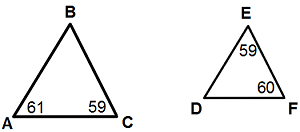#### Question 3 3. If angle a is 30 degrees and angle d is 70 degrees, what is the measure of angle b?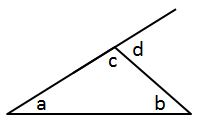#### Question 5 5. Identify x and y in the pictured triangle.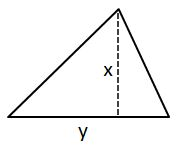### Page 2

#### Question 7 7. In the figure below, Angle 7 = 15x and Angle 10 = 9x + 30. Determine the measure of Angle 10.#### Question 8 8. In the pictured triangle, what is the centroid?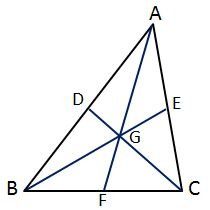#### Question 9 9. If angle a is 66 degrees and angle b is 54 degrees, what is the measure of angle d?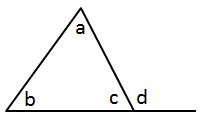#### Question 10 10. What is the area of the pictured rectangle?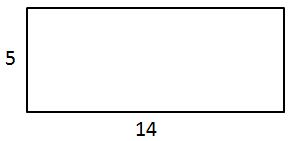### Page 3

#### Question 11 11. Which statement is true regarding areas based on the pictured triangle? Point G is the centroid.#### Question 13 13. If angle a is 68 degrees and angle b is 37 degrees, what is the measure of angle c?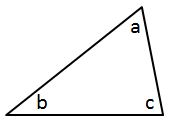#### Question 15 15. In the pictured triangle, what is side B, relative to angle x?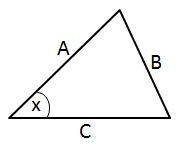### Page 4

#### Question 16 16. What type of triangle is pictured?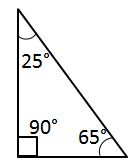#### Question 17 17. Josh claims that these triangles are similar by SSS. Do you agree or disagree with Josh?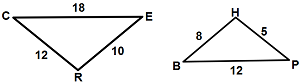#### Question 18 18. In the pictured triangle, identify the altitude(s).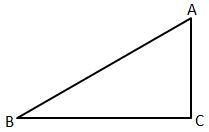#### Question 20 20. In the pictured triangle, what is side A, relative to angle x?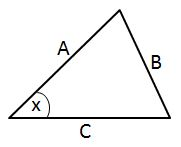### Page 5

#### Question 21 21. What type of line segment is represented by the vertical dotted line in the pictured triangle?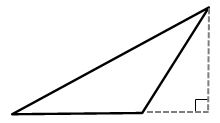#### Question 22 22. Conclude whether triangle BEN is similar to triangle MAT.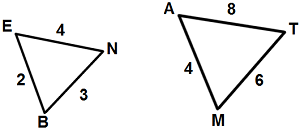#### Question 23 23. If angle a is 60 degrees, what is the measure of angle b?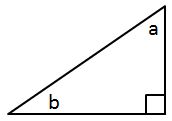### Page 6

#### Question 27 27. Which statement is true regarding areas based on the pictured triangle? Point G is the centroid.#### Question 29 29. What is the area of the pictured triangle?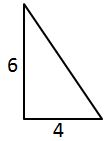#### Question 30 30. In triangle GBN, Angle G is seven more than three times a number, Angle B is five more than four times the number, and Angle N is twelve less than five times the number. Solve for the measure of Angle G.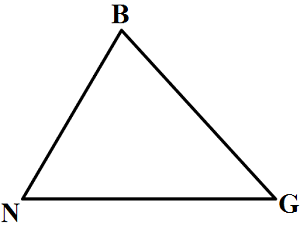#### Properties of Triangles: Homework Help Chapter Exam Instructions

Choose your answers to the questions and click 'Next' to see the next set of questions. You can skip questions if you would like and come back to them later with the yellow "Go To First Skipped Question" button. When you have completed the practice exam, a green submit button will appear. Click it to see your results. Good luck!

Support